Mechanical Engineering - ME 2016 GATE Paper (Practice Test)

# Mechanical Engineering - ME 2016 GATE Paper (Practice Test)

Test Description

## 65 Questions MCQ Test GATE Mechanical (ME) 2023 Mock Test Series | Mechanical Engineering - ME 2016 GATE Paper (Practice Test)

Mechanical Engineering - ME 2016 GATE Paper (Practice Test) for Mechanical Engineering 2023 is part of GATE Mechanical (ME) 2023 Mock Test Series preparation. The Mechanical Engineering - ME 2016 GATE Paper (Practice Test) questions and answers have been prepared according to the Mechanical Engineering exam syllabus.The Mechanical Engineering - ME 2016 GATE Paper (Practice Test) MCQs are made for Mechanical Engineering 2023 Exam. Find important definitions, questions, notes, meanings, examples, exercises, MCQs and online tests for Mechanical Engineering - ME 2016 GATE Paper (Practice Test) below.
Solutions of Mechanical Engineering - ME 2016 GATE Paper (Practice Test) questions in English are available as part of our GATE Mechanical (ME) 2023 Mock Test Series for Mechanical Engineering & Mechanical Engineering - ME 2016 GATE Paper (Practice Test) solutions in Hindi for GATE Mechanical (ME) 2023 Mock Test Series course. Download more important topics, notes, lectures and mock test series for Mechanical Engineering Exam by signing up for free. Attempt Mechanical Engineering - ME 2016 GATE Paper (Practice Test) | 65 questions in 180 minutes | Mock test for Mechanical Engineering preparation | Free important questions MCQ to study GATE Mechanical (ME) 2023 Mock Test Series for Mechanical Engineering Exam | Download free PDF with solutions
 1 Crore+ students have signed up on EduRev. Have you?
Mechanical Engineering - ME 2016 GATE Paper (Practice Test) - Question 1

### Q. 1 – Q. 5 carry one mark each Q. Which of the following is CORRECT with respect to grammar and usage? Mount Everest is ______

Detailed Solution for Mechanical Engineering - ME 2016 GATE Paper (Practice Test) - Question 1

Before superlative article ‘the’ has to be used. “One of” the expression should take plural noun and so option ‘C’ and ‘D’ can’t be the answer.

Mechanical Engineering - ME 2016 GATE Paper (Practice Test) - Question 2

### The policeman asked the victim of a theft, “What did you __________ ?”

Detailed Solution for Mechanical Engineering - ME 2016 GATE Paper (Practice Test) - Question 2

‘lose’ is verb

Mechanical Engineering - ME 2016 GATE Paper (Practice Test) - Question 3

### Despite the new medicine’s ______in treating diabetes, it is not ________ widely

Detailed Solution for Mechanical Engineering - ME 2016 GATE Paper (Practice Test) - Question 3

‘effectiveness’ is noun and ‘prescribed’ is verb. These words are apt and befitting with the word ‘medicine’.

Mechanical Engineering - ME 2016 GATE Paper (Practice Test) - Question 4

Michael lives 10 km away from where I live. Ahmed lives 5 km away and Susan lives 7 km away from where I live. Arun is farther away than Ahmed but closer than Susan from where I live. From the information provided here, what is one possible distance (in km) at which I live from Arun’s place?

Mechanical Engineering - ME 2016 GATE Paper (Practice Test) - Question 5

Michael lives 10 km away from where I live. Ahmed lives 5 km away and Susan lives 7km away from where I live Arun is farther away than Ahmed but closer than susan from where I live. From the information provided here, what is one possible distance (in km) at which I live from Arun’s place?

Detailed Solution for Mechanical Engineering - ME 2016 GATE Paper (Practice Test) - Question 5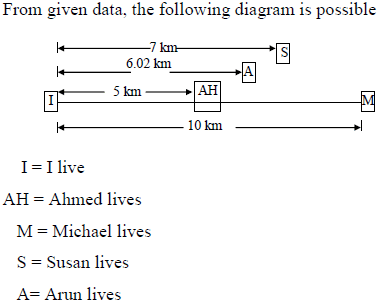Mechanical Engineering - ME 2016 GATE Paper (Practice Test) - Question 6

Q. 6 – Q. 10 carry two marks each.

Q. A person moving through a tuberculosis prone zone has a 50% probability of becoming infected. However only 30% of infected people develop the disease. What percentage of people moving through a tuberculosis prone zone remains infected but does not show symptoms of disease?

Detailed Solution for Mechanical Engineering - ME 2016 GATE Paper (Practice Test) - Question 6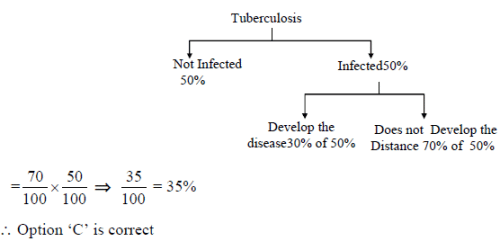Mechanical Engineering - ME 2016 GATE Paper (Practice Test) - Question 7

In a world filled with uncertainty he was glad to have many good friends. He had always assisted them in times of need and was confident that they would reciprocate. However, the events of the last week proved him wrong?
Which of the following inference (s) is/are logically valid and can be inferred from the above passage?
(i) His friends were always asking him to help them
(ii) He felt that when in need of help, his friends would let him down
(iii) He was sure that his friends would help him when in need
(iv) His friends did not help him last week

Detailed Solution for Mechanical Engineering - ME 2016 GATE Paper (Practice Test) - Question 7

The words ‘was confident that they would reciprocate’ and ‘last week proved him wrong’ lead to statements iii and iv as logically valid inferences.

Mechanical Engineering - ME 2016 GATE Paper (Practice Test) - Question 8

Leela is older than her cousin Pavithra. Pavithra’s brother Shiva is older than Leela. When Pavithra and Shiva are visiting Leela, all three like to play chess. Pavithra wins often than Leela does.
Which one of the following statements must be TRUE based on the above?

Detailed Solution for Mechanical Engineering - ME 2016 GATE Paper (Practice Test) - Question 8

From given data, the following arrangement is possible
Shiva
Leela
Pavithra
Among four alternatives, option D is TRUE.

Mechanical Engineering - ME 2016 GATE Paper (Practice Test) - Question 9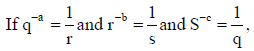the value of abc is______

Detailed Solution for Mechanical Engineering - ME 2016 GATE Paper (Practice Test) - Question 9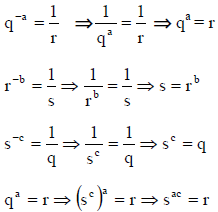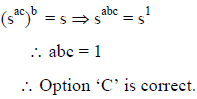Mechanical Engineering - ME 2016 GATE Paper (Practice Test) - Question 10

P,Q,R and S are working on a project. Q can finish the task in 25 days, working alone for 12 hours a day. R can finish the task in 50 days, working along for 12 hours per day. Q worked 12 hours a day but took sick leave in the beginning for two days. R worked 18 hours a day on all days. What is the ratio of work done by Q and R after 7 days from the start of the project?

Detailed Solution for Mechanical Engineering - ME 2016 GATE Paper (Practice Test) - Question 10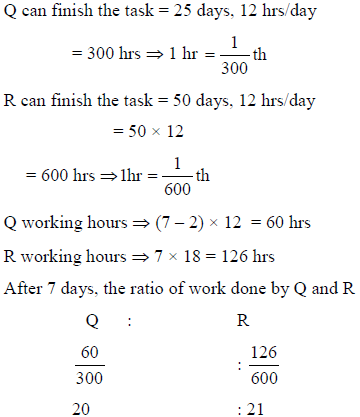Mechanical Engineering - ME 2016 GATE Paper (Practice Test) - Question 11

Q. 11 – Q. 35 carry one mark each:

Q. The solution to the system of equations is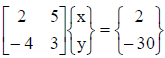Detailed Solution for Mechanical Engineering - ME 2016 GATE Paper (Practice Test) - Question 11

x = 6, y = –2 is the solution of equation

2x+5y = 2 and –4x + 3y = –30

Mechanical Engineering - ME 2016 GATE Paper (Practice Test) - Question 12

If f(t) is a function defined for all t > 0, its laplace transform F(s) is defined as

Detailed Solution for Mechanical Engineering - ME 2016 GATE Paper (Practice Test) - Question 12

By the definition of Laplace transform of f(t)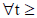, we have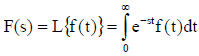Mechanical Engineering - ME 2016 GATE Paper (Practice Test) - Question 13

f(z) = u(x,y) + i v(x,y) is an analytic function of complex variable z = x + iy where i =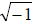. If u(x,y) = 2 xy, then v(x,y) may be expressed as

Detailed Solution for Mechanical Engineering - ME 2016 GATE Paper (Practice Test) - Question 13

Given u =2xy, v = ?
The Cauchy-Riemann equation
ux = vy & vx = – uy are satisfying with option (a) –x2+ y2 + constant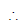V(x,y) = –x2 + y+ constant

Mechanical Engineering - ME 2016 GATE Paper (Practice Test) - Question 14

Consider a Poisson distribution for the tossing of a biased coin. The mean for this distribution is μ. The standard deviation for this distribution is given by

Detailed Solution for Mechanical Engineering - ME 2016 GATE Paper (Practice Test) - Question 14

For Poisson distribution mean = variance
given mean = μvariance = μstandard deviation =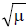*Answer can only contain numeric values
Mechanical Engineering - ME 2016 GATE Paper (Practice Test) - Question 15

Solve the equation x = 10 cos (x) using the Newton-Raphson method. The initial guess is x = π/4.
The value of the predicted root after the first iteration, up to second decimal, is __________.

(Important - Enter only the numerical value in the answer)

Detailed Solution for Mechanical Engineering - ME 2016 GATE Paper (Practice Test) - Question 15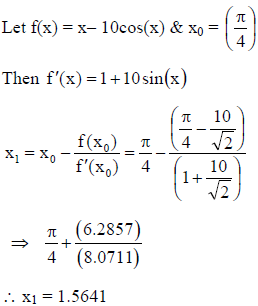*Answer can only contain numeric values
Mechanical Engineering - ME 2016 GATE Paper (Practice Test) - Question 16

A rigid ball of weight 100 N is suspended with the help of a string. The ball is pulled by a horizontal force F such that the string makes an angle of 30? with the vertical. The magnitude of force F (in N) is _____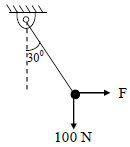Detailed Solution for Mechanical Engineering - ME 2016 GATE Paper (Practice Test) - Question 16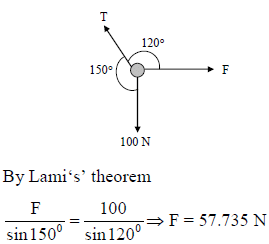Mechanical Engineering - ME 2016 GATE Paper (Practice Test) - Question 17

A point mass M is released from rest and slides down a spherical bowl (of radius R ) from a height H as shown in the figure below. The surface of the bowl is smooth (no friction). The velocity of the mass at the bottom of the bowl is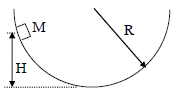Detailed Solution for Mechanical Engineering - ME 2016 GATE Paper (Practice Test) - Question 17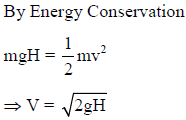Mechanical Engineering - ME 2016 GATE Paper (Practice Test) - Question 18

The cross sections of two hollow bars made of the same material are concentric circles as shown in the figure. It is given that r3 > r1 and r4 > r2, and that the areas of the cross-sections are the same. J1 and J2 are the torsional rigidities of the bars on the left and right, respectively. The ratio J2/J1 is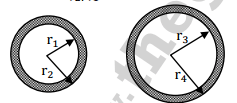Detailed Solution for Mechanical Engineering - ME 2016 GATE Paper (Practice Test) - Question 18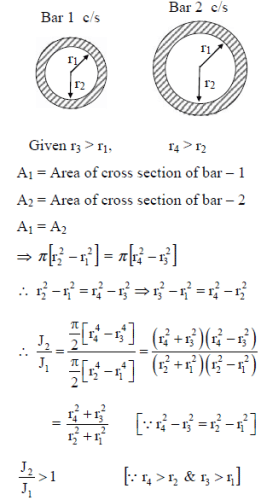Mechanical Engineering - ME 2016 GATE Paper (Practice Test) - Question 19

A cantilever beam having square cross-section of side a is subjected to an end load. If a is increased by 19%, the tip deflection decreases approximately by

Detailed Solution for Mechanical Engineering - ME 2016 GATE Paper (Practice Test) - Question 19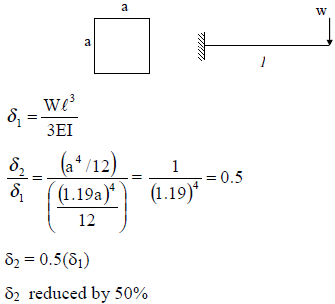*Answer can only contain numeric values
Mechanical Engineering - ME 2016 GATE Paper (Practice Test) - Question 20

A car is moving on a curved horizontal road of radius 100 m with a speed of 20 m/s. The rotating masses of the engine have an angular speed of 100 rad/s in clockwise direction when viewed from the front of the car. The combined moment of inertia of the rotating masses is 10 kg-m2. The magnitude of the gyroscopic moment (in N-m) is_____

Detailed Solution for Mechanical Engineering - ME 2016 GATE Paper (Practice Test) - Question 20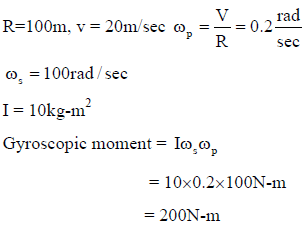*Answer can only contain numeric values
Mechanical Engineering - ME 2016 GATE Paper (Practice Test) - Question 21

A single degree of freedom spring mass system with viscous damping has a spring constant of 10 kN/m. The system is excited by a sinusoidal force of amplitude 100 N. If the damping factor (ratio) is 0.25, the amplitude of steady state oscillation at resonance is ________mm

Detailed Solution for Mechanical Engineering - ME 2016 GATE Paper (Practice Test) - Question 21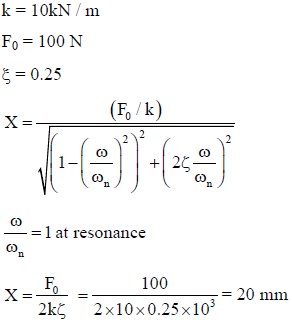Mechanical Engineering - ME 2016 GATE Paper (Practice Test) - Question 22

The spring constant of a helical compression spring DOES NOT depend on

Mechanical Engineering - ME 2016 GATE Paper (Practice Test) - Question 23

The instantaneous stream-wise velocity of a turbulent flow is given as follows: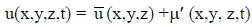The time – average of the fluctuating velocity u' (x,y, z, t)

Detailed Solution for Mechanical Engineering - ME 2016 GATE Paper (Practice Test) - Question 23

Time average of fluctuating velocity is zero.

Mechanical Engineering - ME 2016 GATE Paper (Practice Test) - Question 24

For a floating body, buoyant force acts at the

Detailed Solution for Mechanical Engineering - ME 2016 GATE Paper (Practice Test) - Question 24

For floating body Buoyancy force acts through the centre of buoyancy which is C.G for displaced volume.

Mechanical Engineering - ME 2016 GATE Paper (Practice Test) - Question 25

A plastic sleeve of outer radius ro = 1 mm covers a wire (radius r = 0.5 mm) carrying electric current. Thermal conductivity of the plastic is 0.15 W/m-K. The heat transfer coefficient on the outer surface of the sleeve exposed to air is 25 W/m2-K. Due to the addition of the plastic cover, the heat transfer from the wire to the ambient will

Detailed Solution for Mechanical Engineering - ME 2016 GATE Paper (Practice Test) - Question 25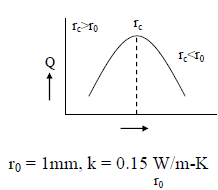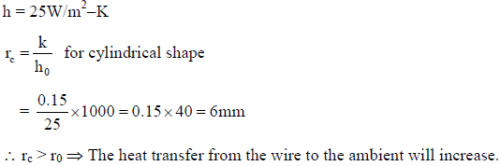Mechanical Engineering - ME 2016 GATE Paper (Practice Test) - Question 26

Which of the following statements are TRUE with respect to heat and work?
(i) They are boundary phenomena
(ii) They are exact differentials
(iii) They are path functions

*Answer can only contain numeric values
Mechanical Engineering - ME 2016 GATE Paper (Practice Test) - Question 27

Propane (C3H8) is burned in an oxygen atmosphere with 10% deficit oxygen with respect to the stoichiometric requirement. Assuming no hydrocarbons in the products, the volume percentage of CO in the products is ____

(Important -  Enter only the numerical value in the answer)

Detailed Solution for Mechanical Engineering - ME 2016 GATE Paper (Practice Test) - Question 27

C3H8 + xO2 → aCO2 + bH2O
Carbon balance :
a = 3
hydrogen balance:
2b = 8 → b = 4
Oxygen balance:
2x = 2a + b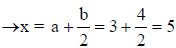For chemically correct or stoichiometric burning, no. of moles of O2 required are = 5.
As it is burnt with 10% deficient oxygen, it will generate CO.
The new equation is
C3H8 + 0.9 x 5O2 → aCO2 + bCO + cH2O
Carbon balance:
a + b = 3
Hydrogen balance:
2c = 8 → c = 4
Oxygen balance:
2a + b + c = 0.9 x 5 x 2 = 9
2a + b + c = 9
⇒ 2a + b + 4 = 9 ⇒ 2a + b = 5 … (1)
a + b = 3 … (2)
By solving (1) & (2)
a = 2 & b = 1
in the exhaust products the no. of moles of CO are 1.% by volume of CO in exhaust.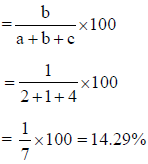*Answer can only contain numeric values
Mechanical Engineering - ME 2016 GATE Paper (Practice Test) - Question 28

Consider two hydraulic turbines identical specific speed and effective head at the inlet. If the speed
ratio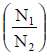of the two turbines is 2, then the respective power ratio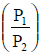_________.

Detailed Solution for Mechanical Engineering - ME 2016 GATE Paper (Practice Test) - Question 28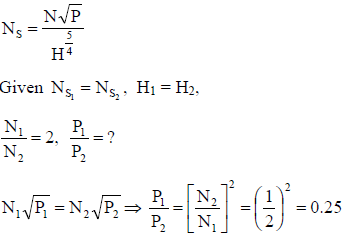Mechanical Engineering - ME 2016 GATE Paper (Practice Test) - Question 29

The INCORRECT statement about regeneration in vapor power cycle is that

Mechanical Engineering - ME 2016 GATE Paper (Practice Test) - Question 30

The “Jominy test” is used to find

Detailed Solution for Mechanical Engineering - ME 2016 GATE Paper (Practice Test) - Question 30

The depth upto which the required hardness is obtained is called as hardenability and it is
determined by using jomney end quench test.

Mechanical Engineering - ME 2016 GATE Paper (Practice Test) - Question 31

Under optimal conditons of the process the temperatures experienced by a copper work piece in fusion welding, brazing and soldering are such that

Detailed Solution for Mechanical Engineering - ME 2016 GATE Paper (Practice Test) - Question 31

In welding ( Fusion welding for melting the parent material the temperature should be greater than the MP of the metal hence it is high. Whereas brazing and soldering are the nonfusion welding operations hence the temperature should be less than the MP of the metal. Brazing temp is above 4270C and soldering is below 4270C

Mechanical Engineering - ME 2016 GATE Paper (Practice Test) - Question 32

The part of a gating system which regulates the rate of pouring of molten metal is

Detailed Solution for Mechanical Engineering - ME 2016 GATE Paper (Practice Test) - Question 32

Rate of pouring of molten metal depends on the flow rate of molten metal. This depends on the choke area and it is the minimum area out of the cross sectional areas of sprue, runner and ingate.

Mechanical Engineering - ME 2016 GATE Paper (Practice Test) - Question 33

The non-traditional machining process that essentially requires vacuum is

Detailed Solution for Mechanical Engineering - ME 2016 GATE Paper (Practice Test) - Question 33

Electron beam machining is the only method carried out under vacuum, to avoid the dispersion of electrons after the magnetic deflector.

*Answer can only contain numeric values
Mechanical Engineering - ME 2016 GATE Paper (Practice Test) - Question 34

In an orthogonal cutting process the tool used has rake angle of zero degree. The measured cutting force and thrust force are 500 N and 250 N, respectively. The coefficient of friction between the tool and the chip is _________

Detailed Solution for Mechanical Engineering - ME 2016 GATE Paper (Practice Test) - Question 34

Because the rake angle is zero, F= Friction force = Fc = Cutting force = 500N, N = Normal to friction force = Ft = Thrust force = 250N,

Coefficient of friction = F/N = 250 / 500 = 0.5

Mechanical Engineering - ME 2016 GATE Paper (Practice Test) - Question 35

Match the following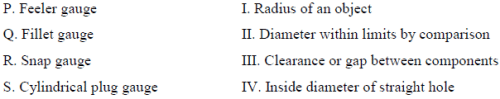Detailed Solution for Mechanical Engineering - ME 2016 GATE Paper (Practice Test) - Question 35

Feeler gauge is used for checking the clearance or gap between the parts, radius is checked by fillet gauge, limits of diameter of shaft is checked by snap gauge and plug gauge is used for checking the diameter of hole.

*Answer can only contain numeric values
Mechanical Engineering - ME 2016 GATE Paper (Practice Test) - Question 36

Q.36 to 65 (Two marks Questions)

Q. Consider the function f(x) = 2x3 -3x2 in the domain [-1, 2]. The global minimum of f(x) is ____

(Important - Enter only the numerical value in the answer)

Detailed Solution for Mechanical Engineering - ME 2016 GATE Paper (Practice Test) - Question 36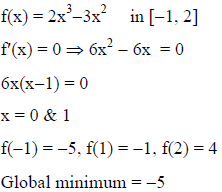*Answer can only contain numeric values
Mechanical Engineering - ME 2016 GATE Paper (Practice Test) - Question 37

If y = f(x) satisfies the boundary value problem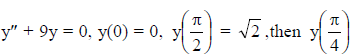is ________.

Detailed Solution for Mechanical Engineering - ME 2016 GATE Paper (Practice Test) - Question 37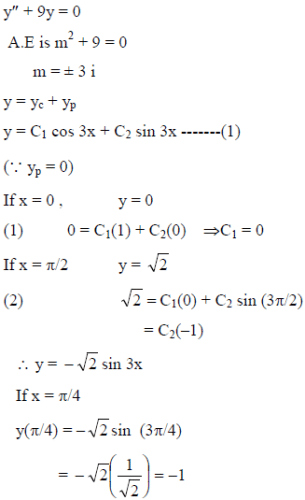Mechanical Engineering - ME 2016 GATE Paper (Practice Test) - Question 38

The value of the integral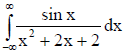evaluated using contour integration and the residue theorem is

Detailed Solution for Mechanical Engineering - ME 2016 GATE Paper (Practice Test) - Question 38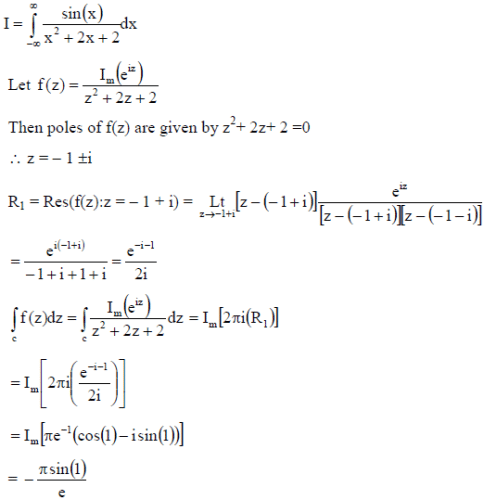*Answer can only contain numeric values
Mechanical Engineering - ME 2016 GATE Paper (Practice Test) - Question 39

Gauss-Seidel method is used to solve the following equations (as per the given order).
x1 + 2x2 + 3x3 = 5
2x1 + 3x2 + x3 = 1
3x1 + 2x2 + x3 = 3
Assuming initial guess as x1 = x2 = x3 = 0, the value of x3 after the first iteration is ________

(Important - Enter only the numerical value in the answer)

Detailed Solution for Mechanical Engineering - ME 2016 GATE Paper (Practice Test) - Question 39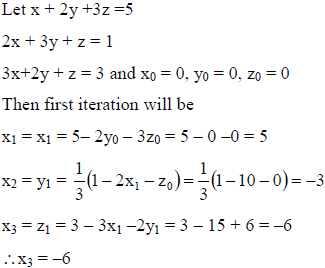*Answer can only contain numeric values
Mechanical Engineering - ME 2016 GATE Paper (Practice Test) - Question 40

A block of mass m rests on an inclined plane and is attached by a string to the wall as shown in the figure. The coefficient of static friction between the plane and the block is 0.25. The string can withstand a maximum force of 20 N. The maximum value of the mass (m) for which the string will not break and the block will be in static equilibrium is ___kg.
Take cosθ = 0.8 and sin θ = 0.6.
Acceleraiton due to gravity g = 10 m/s2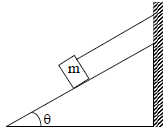Detailed Solution for Mechanical Engineering - ME 2016 GATE Paper (Practice Test) - Question 40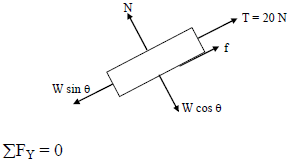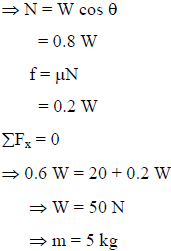Mechanical Engineering - ME 2016 GATE Paper (Practice Test) - Question 41

A two – member truss PQR is supporting a load W. The axial forces in members PQ and QR are respectively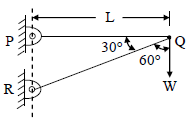Detailed Solution for Mechanical Engineering - ME 2016 GATE Paper (Practice Test) - Question 41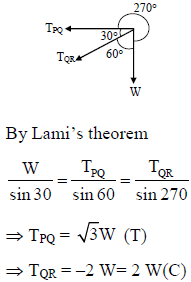*Answer can only contain numeric values
Mechanical Engineering - ME 2016 GATE Paper (Practice Test) - Question 42

A horizontal bar with a constant cross-section is subjected to loading as shown in the figure. The Young’s moduli for the sections AB and BC are 3E and E, respectively.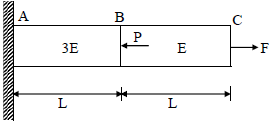For the deflection at C to be zero, the ratio P/F is ____

(Important - Enter only the numerical value in the answer)

Detailed Solution for Mechanical Engineering - ME 2016 GATE Paper (Practice Test) - Question 42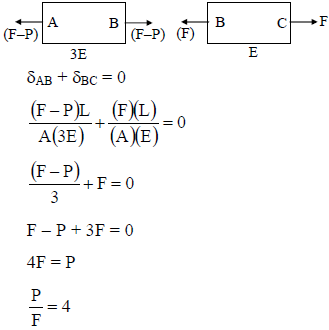*Answer can only contain numeric values
Mechanical Engineering - ME 2016 GATE Paper (Practice Test) - Question 43

The figure shows cross-section of a beam subjected to bending. The area moment of inertia (in mm4) of this cross-section about its base is ________

(Important -  Enter only the numerical value in the answer)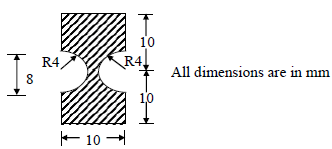Detailed Solution for Mechanical Engineering - ME 2016 GATE Paper (Practice Test) - Question 43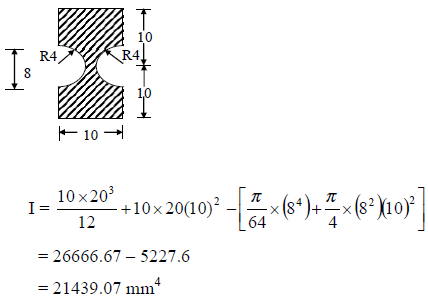*Answer can only contain numeric values
Mechanical Engineering - ME 2016 GATE Paper (Practice Test) - Question 44

A simply-supported beam of length 3L is subjected to the loading shown in the figure.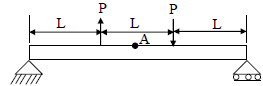It is given that P = 1 N, L = 1 m and Young’s modulus E = 200 GPa. The cross-section is a square with dimension 100 mm. The bending stress (in Pa) at the point A located at the top surface of the beam at a distance of 1.5L from the left end is (Indicate compressive stress by a negative sign and tensile stress by a positive sign).

(Important - Enter only the numerical value in the answer)

Detailed Solution for Mechanical Engineering - ME 2016 GATE Paper (Practice Test) - Question 44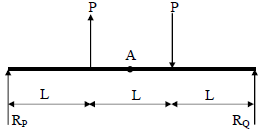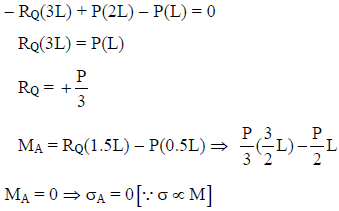*Answer can only contain numeric values
Mechanical Engineering - ME 2016 GATE Paper (Practice Test) - Question 45

A slider crank mechanism with crank radius 200 mm and connecting rod length 800 mm is shown. The crank is rotating at 600 rpm in the counterclockwise direction. In the configuration shown, the crank makes an angle of 90o with the sliding direction of the slider, and a force of 5 kN is acting on the slider. Neglecting the inertia forces, the turning moment on the crank (in kN-m) is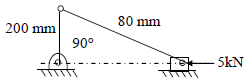(Important - Enter only the numerical value in the answer)

Detailed Solution for Mechanical Engineering - ME 2016 GATE Paper (Practice Test) - Question 45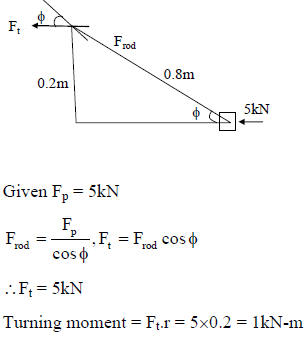Mechanical Engineering - ME 2016 GATE Paper (Practice Test) - Question 46

In the gear train shown, gear 3 is carried on arm 5. Gear 3 meshes with gear 2 and gear 4. The number of teeth on gear 2,3, and 4 are 60, 20, and 100, respectively. If gear 2 is fixed and gear 4 rotates with an angular velocity of 100 rpm in the counterclockwise direction, the angular speed of arm 5 (in rpm) is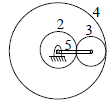Detailed Solution for Mechanical Engineering - ME 2016 GATE Paper (Practice Test) - Question 46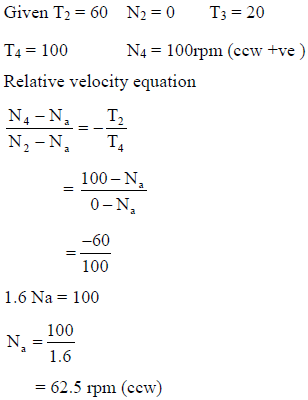Mechanical Engineering - ME 2016 GATE Paper (Practice Test) - Question 47

A solid disc with radius a is connected to a spring at a point d above center of the disc. The other end of the spring is fixed to the vertical wall. The disc is free to roll without slipping on the ground. The mass of the disc is M and the spring constant is K. The polar moment of inertia for the disc about its centere is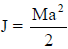The natural frequency of this system in rad/s is given by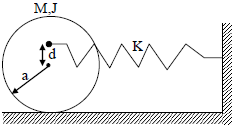Detailed Solution for Mechanical Engineering - ME 2016 GATE Paper (Practice Test) - Question 47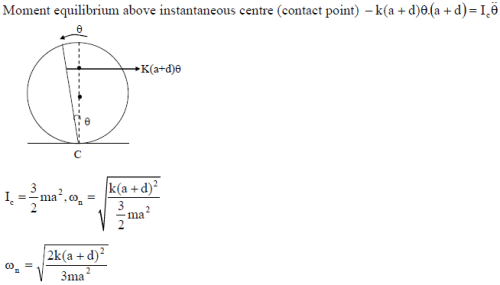Mechanical Engineering - ME 2016 GATE Paper (Practice Test) - Question 48

The principal stresses at a point inside a solid object are σ1 = 100 MPa, σ2 = 100 MPa and σ3 = 0 MPa. The yield strength of the material is 200 MPa. The factor of safety calculated using Tresca (maximum shear stress) theory is nT and the factor of safety calculated using von Mises (maximum distortional energy) theory is nv. Which one of the following relations is TRUE?

Detailed Solution for Mechanical Engineering - ME 2016 GATE Paper (Practice Test) - Question 48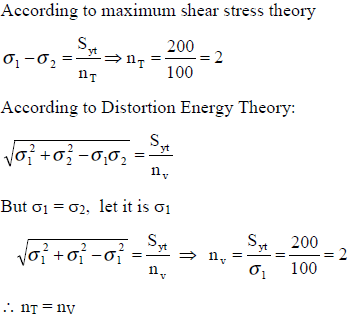*Answer can only contain numeric values
Mechanical Engineering - ME 2016 GATE Paper (Practice Test) - Question 49

An inverted U-tube manometer is used to measure the pressure difference between two pipes A and B, as shown in the figure. Pipe A is carrying oil (Specific gravity = 0.8) and Pipe B is carrying water. The densities of air and water are 1.16 kg/m and 1000 kg/m3, respectively. The pressure difference between pipes A and B is _______kPa.
Acceleration due to gravity g = 10 m/s2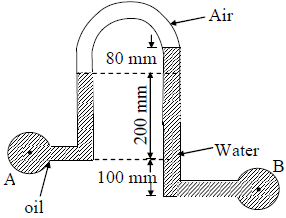(Important - Enter only the numerical value in the answer)

Detailed Solution for Mechanical Engineering - ME 2016 GATE Paper (Practice Test) - Question 49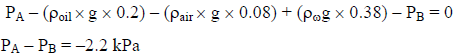*Answer can only contain numeric values
Mechanical Engineering - ME 2016 GATE Paper (Practice Test) - Question 50

Oil (kinematic viscosity, νoil = 1.0 x 10-5 m2/s) flows through a pipe of 0.5 m diameter with velocity of 10 m/s. Water (Kinematic viscosity, νw = 0.89 x 10-6 m2/s) is flowing through a model pipe of diameter 20 mm. For satisfying the dynamic similarity, the velocity of water (in m/s) is ____

(Important - Enter only the numerical value in the answer)

Detailed Solution for Mechanical Engineering - ME 2016 GATE Paper (Practice Test) - Question 50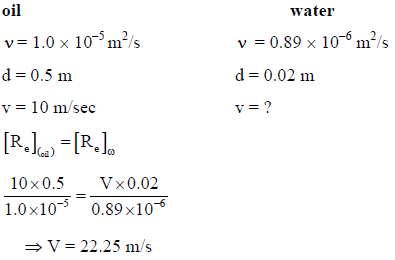*Answer can only contain numeric values
Mechanical Engineering - ME 2016 GATE Paper (Practice Test) - Question 51

A steady laminar boundary layer is formed over a flat plate as shown in the figure. The free stream velocity of the fluid is Uo . The velocity profile at the inlet a-b is uniform, while that at a downstream location c-d is given by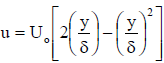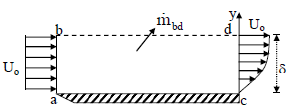The ratio of the mass flow rate, bd, mbd leaving through the horizontal section b-d to that entering through the vertical section a-b is

(Important - Enter only the numerical value in the answer)

Detailed Solution for Mechanical Engineering - ME 2016 GATE Paper (Practice Test) - Question 51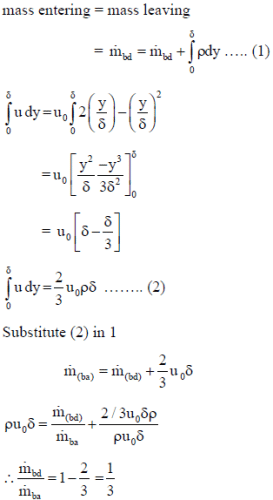*Answer can only contain numeric values
Mechanical Engineering - ME 2016 GATE Paper (Practice Test) - Question 52

A steel ball of 10 mm diameter at 1000 K is required to be cooled to 350 K by immersing it in a water environment at 300 K. The convective heat transfer coefficient is 1000 W/m2-K. Thermal conductivity of steel is 40 W/m-K.The time constant for the cooling process τ is 16s. The time required (in s) to reach the final temperature is________

(Important - Enter only the numerical value in the answer)

Detailed Solution for Mechanical Engineering - ME 2016 GATE Paper (Practice Test) - Question 52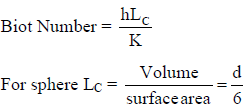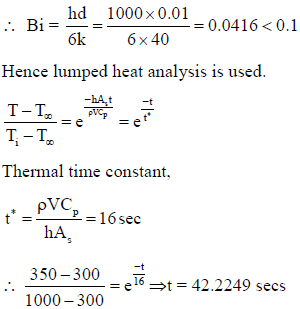*Answer can only contain numeric values
Mechanical Engineering - ME 2016 GATE Paper (Practice Test) - Question 53

An infinitely long furnace of 0.5 m x 0.4 m cross-section is shown in the figure below. Consider all surfaces of the furnace to be black. The top and bottom walls are maintained at temperature T1 = T3 = 927oC while the side walls are at temperature T2 = T4 = 527oC. The view factor, F1-2 is 0.26. The net radiation heat loss or gain on side 1 is _____ W/m.

Stefan-Boltzman constant = 5.67 x 10-8 W/m2-K4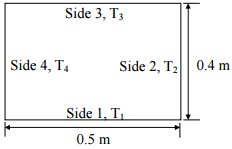(Important - Enter only the numerical value in the answer)

Detailed Solution for Mechanical Engineering - ME 2016 GATE Paper (Practice Test) - Question 53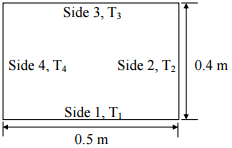T1 = 927oC = 1200K,

T2 = 527oC = 800K

F12 = F14 = 0.26

F11 + F12 + F13 + F14 = 1

F13 = 0.48

Q = Q12 + Q13 + Q14

Q13 = 0 since the temperatures are same

Q = Q12 + Q14 = 2×σb×A×F12(T14 - T24)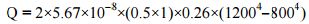= 24530.688 Watt

*Answer can only contain numeric values
Mechanical Engineering - ME 2016 GATE Paper (Practice Test) - Question 54

A fluid (Prandtl number, Pr = 1) at 500 K flows over a flat plate of 1.5 m length, maintained at 300 K. The velocity of the fluid is 10 m/s. Asuming kinematic viscosity, v = 30 x 10-6 m2/s, the thermal boundary layer thickness (in mm) at 0.5 m from the leading edge is ____

(Important - Enter only the numerical value in the answer)

Detailed Solution for Mechanical Engineering - ME 2016 GATE Paper (Practice Test) - Question 54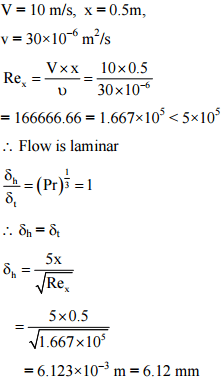*Answer can only contain numeric values
Mechanical Engineering - ME 2016 GATE Paper (Practice Test) - Question 55

For water at 25oC, dps/dTs = 0.189 kPa/K (ps is the saturation pressure in kPa and Ts is the saturation temperature in K) and the specific volume of dry saturated vapour is 43.38 m3/kg. Assume that the specific volume of liquid is negligible in comparson with that of vapour. Using the Clausius-Clapeyron equation, an estimate of the enthalpy of evaporation of water at 25oC (in kJ/kg) is ___

(Important - Enter only the numerical value in the answer)

Detailed Solution for Mechanical Engineering - ME 2016 GATE Paper (Practice Test) - Question 55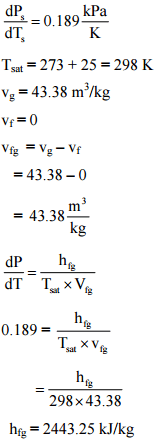*Answer can only contain numeric values
Mechanical Engineering - ME 2016 GATE Paper (Practice Test) - Question 56

An ideal gas undergoes a reversible process in which the pressure varies linearly with volume. The conditions at the start (subscript 1) and at the end (subscript 2) of the process with usual notation are: p1 = 100 kPa, V1 = 0.2 mand p2 = 200 kPa, V2 = 0.1 m3 and the gas constant, R = 0.275 kJ/kg-K. The magnitude of the work required for the process (in kJ) is______

(Important - Enter only the numerical value in the answer)

Detailed Solution for Mechanical Engineering - ME 2016 GATE Paper (Practice Test) - Question 56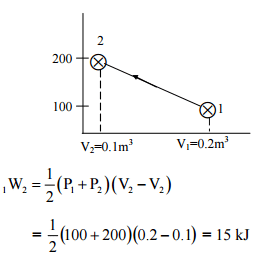*Answer can only contain numeric values
Mechanical Engineering - ME 2016 GATE Paper (Practice Test) - Question 57

In a steam power plant operating on an ideal Rankine cycle, superheated steam enters the turbine at 3 MPa and 350oC. The condenser pressure is 75 kPa. The thermal efficiency of the cycle is _______ percent.

Given data:

For saturated liquid, at P = 75 kPa,

hf = 384.39 kJ/kg, vf = 0.001037 m3/kg,

sf = 1.213 kJ/kg-K

At 75 kPa, hfg = 2278.6 kJ/kg,

sfg = 6.2434 kJ/kg-K

At P = 3 MPa and T = 350o C (Superheated steam), h = 3115.3 kJ/kg,

s = 6.7428 kJ/kg-K

(Important - Enter only the numerical value in the answer)

Detailed Solution for Mechanical Engineering - ME 2016 GATE Paper (Practice Test) - Question 57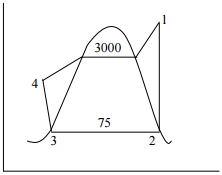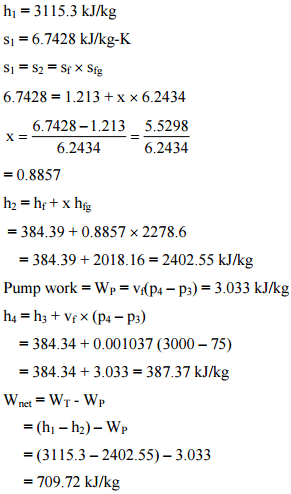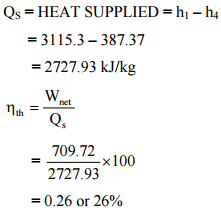*Answer can only contain numeric values
Mechanical Engineering - ME 2016 GATE Paper (Practice Test) - Question 58

A hypothetical engineering stress-strain curve shown in the figure has three straight lines PQ, QR, RS with coordinates P(0,0), Q (0.2,100), R(0.6, 140) and S(0.8, 130). ‘Q’ is the yield point, 'R' is the UTS point and 'S' the fracture point.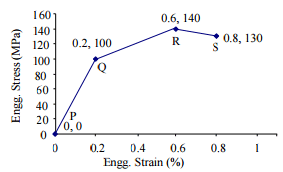The toughness of the material (in MJ/m3) is ____

(Important - Enter only the numerical value in the answer)

Detailed Solution for Mechanical Engineering - ME 2016 GATE Paper (Practice Test) - Question 58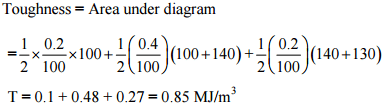*Answer can only contain numeric values
Mechanical Engineering - ME 2016 GATE Paper (Practice Test) - Question 59

Heat is removed from a molten metal of mass 2 kg at a constant rate of 10 kW till it is completely solidified. The cooling curve is shown in the figure.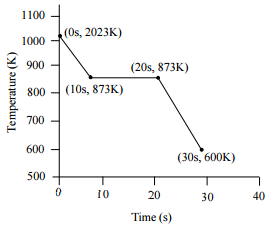Assuming uniform temperature throughout the volume of the metal during solidification, the latent heat of fusion of the metal (in kJ/kg) is ____

Detailed Solution for Mechanical Engineering - ME 2016 GATE Paper (Practice Test) - Question 59

m = 2 kg, Q = 10 kW

time taken for removing latent heat = 20 – 10 = 10 sec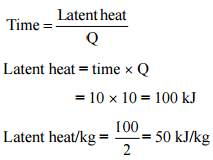Mechanical Engineering - ME 2016 GATE Paper (Practice Test) - Question 60

The too life equation for HSS tool is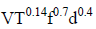= Constant. The tool life (T) of 30 min is obtained using the following cutting conditions = 45 m/min, f = 0.35 mm, d = 2.0 mm. If speed (V), feed (f) and depth of cut (D) are increased individually by 25%, the tool life (in min) is

Detailed Solution for Mechanical Engineering - ME 2016 GATE Paper (Practice Test) - Question 60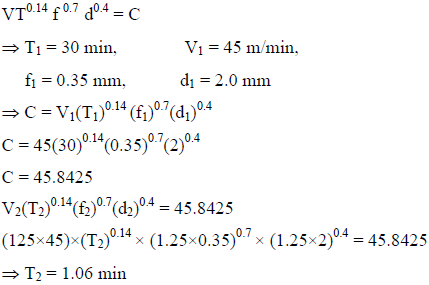Mechanical Engineering - ME 2016 GATE Paper (Practice Test) - Question 61

A cylindrical job with diameter of 200 mm and height of 100 mm is to be cast using modulus method of riser design. Assume that the bottom surface of cylindrical riser does not contribute as cooling surface. If the diameter of the riser is equal to its height, then the height of the riser (in mm) is

Detailed Solution for Mechanical Engineering - ME 2016 GATE Paper (Practice Test) - Question 61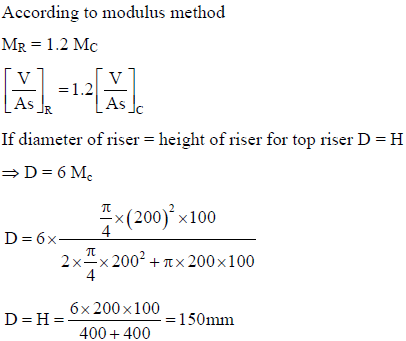*Answer can only contain numeric values
Mechanical Engineering - ME 2016 GATE Paper (Practice Test) - Question 62

A 300 mm thick slab is being cold rolled using roll of 600 mm diameter. If the coefficient of friction is 0.08, the maximum possible reduction (in mm) is ______

(Important - Enter only the numerical value in the answer)

Detailed Solution for Mechanical Engineering - ME 2016 GATE Paper (Practice Test) - Question 62

Maximum possible reduction =

ΔH/ pass = μ2R = 0.082 x 300 = 1.92 mm

Mechanical Engineering - ME 2016 GATE Paper (Practice Test) - Question 63

The figure below represents a triangle PQR with initial coordinates of the vertices as P(1,3), Q (4,5) and R(5,3.5). The triangle is rotated in the X-Y plane about the vertex P by angle θ in clockwise direction. If sin θ = 0.6 and cos θ = 0.8, the new coordinates of the vertex Q are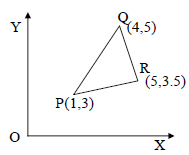Detailed Solution for Mechanical Engineering - ME 2016 GATE Paper (Practice Test) - Question 63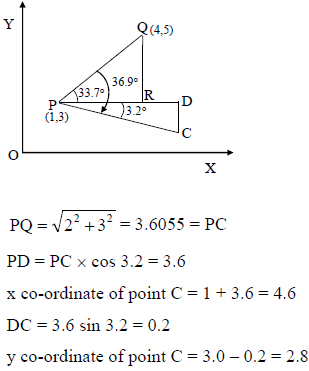*Answer can only contain numeric values
Mechanical Engineering - ME 2016 GATE Paper (Practice Test) - Question 64

The annual demand for an item is 10,000 units. The unit cost is Rs. 100 and inventory carrying charges are 14.4% of the unit cost per annum. The cost of one procurement is Rs. 2000. The time between two consecutive orders to meet the above demand is _____ month (s)

(Important - Enter only the numerical value in the answer)

Detailed Solution for Mechanical Engineering - ME 2016 GATE Paper (Practice Test) - Question 64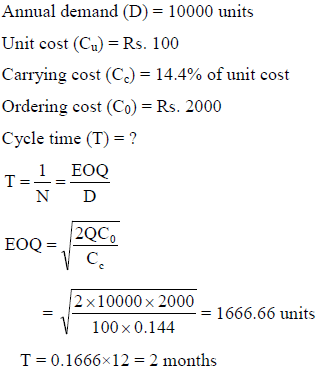Mechanical Engineering - ME 2016 GATE Paper (Practice Test) - Question 65

Maximize Z = 15X1 + 20X2
Subject to
12X1 + 4X2 > 36
12X1 - 6X2 < 24
X1, X2 > 0
The above linear programming problem has

Detailed Solution for Mechanical Engineering - ME 2016 GATE Paper (Practice Test) - Question 65

Max Z = 15x1 + 20x2
Subjected to
12x1 + 4x2 ≥ 36
12x1 - 6x2 ≤ 24
x1, x2 ≥ 0 ,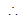unbounded solution.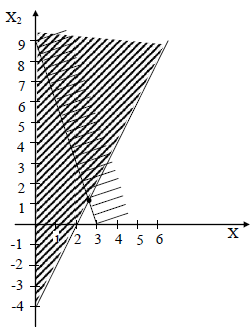## GATE Mechanical (ME) 2023 Mock Test Series

27 docs|243 tests
Information about Mechanical Engineering - ME 2016 GATE Paper (Practice Test) Page
In this test you can find the Exam questions for Mechanical Engineering - ME 2016 GATE Paper (Practice Test) solved & explained in the simplest way possible. Besides giving Questions and answers for Mechanical Engineering - ME 2016 GATE Paper (Practice Test), EduRev gives you an ample number of Online tests for practice

## GATE Mechanical (ME) 2023 Mock Test Series

27 docs|243 tests(Scan QR code)